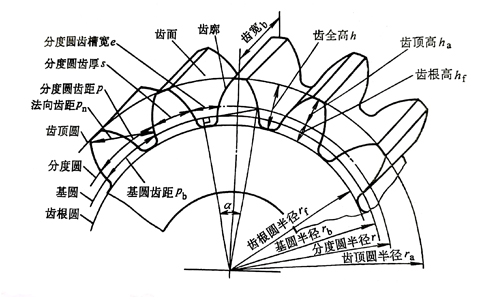• 抖音• 微信0755-82113520、13823217507(微信同号)站点地图|法律申明|English名称 符号 计算公式 小齿轮 大齿轮 模数 m 根据齿轮受力和结构情况需要确定，选取标准值 压力角 a 20° 分度圆直径 d d1=mz1 d2=mz2 齿顶高 ha ha1=ha2=ha* m 齿根高 hf hf1=hf2=(ha*+c*) m 齿全高 h h1=h2=(2ha*+c*) m 齿顶圆直径 da da1=d1+2ha1=(z1+2ha*) m da2=d2±2ha2=(z2±2ha*) m 齿根圆直径 df df1=d1-2hf1=(z1-2ha*-2c*) m df2=d2∓2hf2=（z2∓2ha*∓2c*）m 基圆直径 db db1=mz1cosα db2=mz2cosα 齿距 p p=πm 基圆齿距 pb pb=pcosα 分度圆齿厚 s s=πm/2 分度圆齿槽宽 e e=πm/2 节圆直径 d′ d′=d（当中心距为标准中心距a时） 传动比 i i12=ω1/ω2=d′2/d′1=db2/db1=z2/z1 标准中心距 a a=1/2（d1±d2）=m/2（z1±z2） 顶隙 c c=c * m